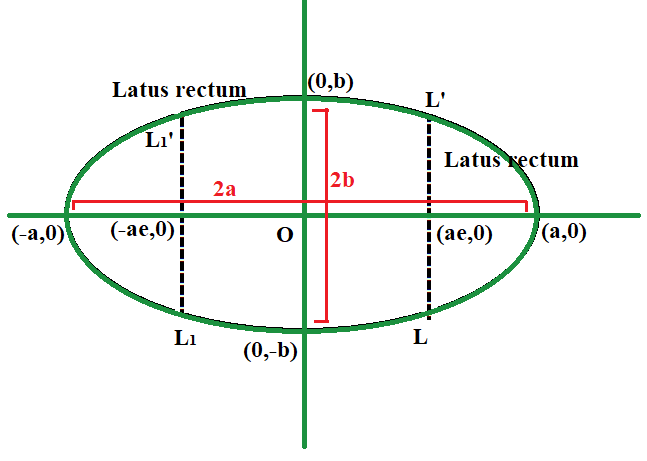# Program to find the Eccentricity of an Ellipse

• Last Updated : 04 May, 2021

Given two positive integers A and B, representing the length of the semi-major and semi-minor axis of an ellipse of the equation, the task is to find the eccentricity of the given ellipse.

Examples:

Input: A = 12, B = 9
Output: 0.66

Input: A = 6, B = 3
Output: 0.87

Approach: The given problem can be solved based on the following formula to calculate the eccentricity of an ellipse which is given by:where,
A = Length of semi major axis
B = Length of semi minor axisTherefore, print the value ofas the eccentricity of the ellipse.

Below is the implementation of the above approach:

## C++

 // C++ program for the above approach #include using namespace std; // Function to find the// eccentricity of ellipsevoid findEccentricity(double A,                      double B){    // Store the squares of length of    // semi-major and semi-minor axis    double semiMajor = A * A;    double semiMinor = B * B;     // Calculate the eccentricity    double ans = sqrt(1 - semiMinor / semiMajor);     // Print the result    cout << fixed << setprecision(2)         << ans;} // Driver Codeint main(){    double A = 12, B = 9;    findEccentricity(A, B);     return 0;}

## Java

 // Java program for the above approachimport java.util.*; class GFG{ // Function to find the// eccentricity of ellipsestatic void findEccentricity(double A, double B){    // Store the squares of length of    // semi-major and semi-minor axis    double semiMajor = A * A;    double semiMinor = B * B;         // Calculate the eccentricity    double ans = Math.sqrt(1 - semiMinor / semiMajor);     // Print the result    System.out.format("%.2f", ans);} // Driver Codepublic static void main(String arr[]){    double A = 12, B = 9;    findEccentricity(A, B);}} // This code is contributed by kirti

## Python3

 # Python3 program for the above approachimport math # Function to find the# eccentricity of ellipsedef findEccentricity(A, B):         # Store the squares of length of    # semi-major and semi-minor axis    semiMajor = A * A    semiMinor = B * B     # Calculate the eccentricity    ans = math.sqrt(1 - semiMinor / semiMajor)     # Print the result    print('%.2f' % ans) # Driver Codeif __name__ == "__main__":     A = 12    B = 9         findEccentricity(A, B)     # This code is contributed by ukasp

## C#

 // C# program for the above approachusing System; class GFG{ // Function to find the// eccentricity of ellipsestatic void findEccentricity(double A, double B){         // Store the squares of length of    // semi-major and semi-minor axis    double semiMajor = A * A;    double semiMinor = B * B;          // Calculate the eccentricity    double ans = Math.Sqrt(1 - semiMinor / semiMajor);      // Print the result    Console.Write(Math.Round(ans, 2));} // Driver codestatic void Main(){    double A = 12, B = 9;         findEccentricity(A, B);}} // This code is contributed by code_hunt

## Javascript

 

Output:

0.66

Time Complexity: O(1)
Auxiliary Space: O(1)

My Personal Notes arrow_drop_up